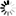# WS4J Demo

WS4J (WordNet Similarity for Java) measures semantic similarity/relatedness between words.

Type in texts below, or use:

 1 Input mode Word    Sentence 2 Sentence 1 3 Sentence 2 4 Submit

## WUP### Latency / Throughput### Description of WUP

This measure calculates relatedness by considering the depths of the two synsets in the WordNet taxonomies, along with the depth of the LCS
WUP(s1, s2) = 2*dLCS.depth / ( min_{dlcs in dLCS}(s1.depth - dlcs.depth)) + min_{dlcs in dLCS}(s2.depth - dlcs.depth) ), where dLCS(s1, s2) = argmax_{lcs in LCS(s1, s2)}(lcs.depth).

### Parameters

• min score = 0.0
• max score = 1.0
• error score = -1.0
• acceptable pos pairs = [['n', 'n'], ['v', 'v']]
• use all senses = true
• use root node = true

## JCN### Latency / Throughput### Description of JCN

Originally a distance measure which also uses the notion of information content, but in the form of the conditional probability of encountering an instance of a child-synset given an instance of a parent synset.
JCN(s1, s2) = 1 / jcn_distance where jcn_distance(s1, s2) = IC(s1) + IC(s2) - 2*IC(LCS(s1, s2)); when it's 0, jcn_distance(s1, s2) = -Math.log_e( (freq(LCS(s1, s2).root) - 0.01D) / freq(LCS(s1, s2).root) ) so that we can have a non-zero distance which results in infinite similarity.

### Parameters

• min score = 0.0
• max score = Infinity
• error score = -1.0
• acceptable pos pairs = [['n', 'n'], ['v', 'v']]
• use all senses = true
• use root node = true

## LCH### Latency / Throughput### Description of LCH

LCH relies on the length of the shortest path between two synsets for their measure of similarity.
LCH(s1, s2) = -Math.log_e( LCS(s1, s2).length / ( 2 * max_depth(pos) ) ).

### Parameters

• min score = 0.0
• max score = Infinity
• error score = -1.0
• acceptable pos pairs = [['n', 'n'], ['v', 'v']]
• use all senses = true
• use root node = true
• max depth N = 20
• max depth V = 14

## LIN### Latency / Throughput### Description of LIN

Idea is similar to JCN with small modification.
LIN(s1, s2) = 2*IC(LCS(s1, s2) / (IC(s1) + IC(s2)).

### Parameters

• min score = 0.0
• max score = 1.0
• error score = -1.0
• acceptable pos pairs = [['n', 'n'], ['v', 'v']]
• use all senses = true
• use root node = true

## RES### Latency / Throughput### Description of RES

Resnik defined the similarity between two synsets to be the information content of their lowest super-ordinate (most specific common subsumer)
RES(s1, s2) = IC(LCS(s1, s2)).

### Parameters

• min score = 0.0
• max score = Infinity
• error score = -1.0
• acceptable pos pairs = [['n', 'n'], ['v', 'v']]
• use all senses = true
• use root node = true

## PATH### Latency / Throughput### Description of PATH

This module computes the semantic relatedness of word senses by counting the number of nodes along the shortest path between the senses in the 'is-a' hierarchies of WordNet.
PATH(s1, s2) = 1 / path_length(s1, s2).

### Parameters

• min score = 0.0
• max score = 1.0
• error score = -1.0
• acceptable pos pairs = [['n', 'n'], ['v', 'v']]
• use all senses = true
• use root node = true

## LESK### Latency / Throughput### Description of LESK

Lesk (1985) proposed that the relatedness of two words is proportional to to the extent of overlaps of their dictionary definitions. This LESK measure is based on adapted Lesk from Banerjee and Pedersen (2002) which uses WordNet as the dictionary for the word definitions. Computational cost is relatively high due to combinations of linked synsets to explore definitions, and need to process these texts.
LESK(s1, s2) = sum_{s1' in linked(s1), s2' in linked(s2)}(overlap(s1'.definition, s2'.definition)).

### Parameters

• min score = 0.0
• max score = Infinity
• error score = -1.0
• acceptable pos pairs = [['a', 'a'], ['a', 'r'], ['a', 'n'], ['a', 'v'], ['r', 'a'], ['r', 'r'], ['r', 'n'], ['r', 'v'], ['n', 'a'], ['n', 'r'], ['n', 'n'], ['n', 'v'], ['v', 'a'], ['v', 'r'], ['v', 'n'], ['v', 'v']]
• use all senses = true
• use stemmer = false
• use stop words = false
• normalize score = false
• word weighting = false

## HSO### Latency / Throughput### Description of HSO

This relatedness measure is based on an idea that two lexicalized concepts are semantically close if their WordNet synsets are connected by a path that is not too long and that "does not change direction too often".
Computational cost is relatively high since recursive search is done on subtrees in the horizontal, upward and downward directions.
HSO(s1, s2) = const_C - path_length(s1, s2) - const_k * num_of_changes_of_directions(s1, s2)

### Parameters

• min score = 0.0
• max score = 16.0
• error score = -1.0
• acceptable pos pairs = [['a', 'a'], ['a', 'r'], ['a', 'n'], ['a', 'v'], ['r', 'a'], ['r', 'r'], ['r', 'n'], ['r', 'v'], ['n', 'a'], ['n', 'r'], ['n', 'n'], ['n', 'v'], ['v', 'a'], ['v', 'r'], ['v', 'n'], ['v', 'v']]
• use all senses = true

Google App Engine Performance Settings:
• Frontend Instance Class: F2 (1200Hz, 256MB)
• Max Idle Instances: 1
• Min Pending Latency: 15.0s

WS4J demo is maintained by Hideki Shima.